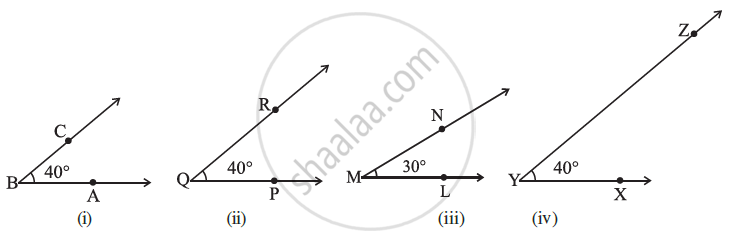# Congruence of Angles:Make a trace-copy of ∠ PQR. Try to superpose it on ∠ABC.
For this, first, place Q on B and QP along with BA.
Thus, ∠ PQR matches exactly with ∠ ABC.
That is, ∠ ABC and ∠ PQR are congruent.
We write ∠ ABC ≅ ∠ PQR or m∠ ABC =m∠ PQR...(In this case, the measure is 40°).

Now, you take a trace-copy of ∠ LMN. Try to superpose it on ∠ ABC.
Place M on B and vec"ML"   "along" vec" BA".
No, in this case, it does not happen. You find that ∠ ABC and ∠ LMN do not cover each other exactly. So, they are not congruent.

Now, observe ∠XYZ and ∠ABC. The rays vec" YX" and vec" YZ", respectively appear to be longer than vec" BA" and vec" BC".
You may, hence, think that ∠ABC is smaller than ∠XYZ. But remember that the rays in the figure only indicate the direction and not any length. On superposition, you will find that these two angles are also congruent.

We write               ∠ABC ≅ ∠XYZ....................(ii)
or                        m∠ABC = m∠XYZ

In view of (i) and (ii), we may even write
∠ ABC ≅ ∠ PQR  ≅ ∠ XYZ

If two angles have the same measure, they are congruent. Also, if two angles are congruent, their measures are the same.

If you would like to contribute notes or other learning material, please submit them using the button below.

### Shaalaa.com

What is Congruence of Angles? [00:08:16]
S
0%